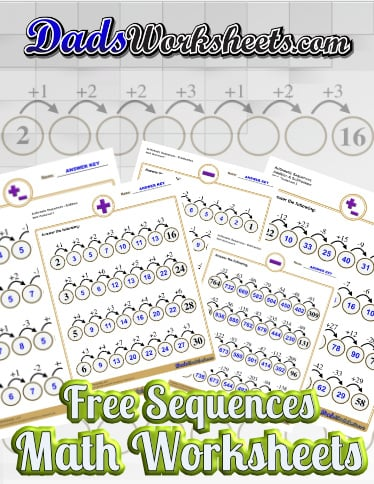# Subtraction Sequences Worksheets

These subtraction worksheets allow students to practice simple sequences of subtraction to arrive at a final answer to a problem. The worksheets start with very small differences and progress through multi-digit subtraction. There are also worksheets that mix addition and subtraction steps in the sequences for practicing both of these arithmetic skills together. They are a fun alternative to simple subtraction problems that keep the subtraction skills moving along!

## Need More Sequences?

Looking for more sequences worksheets? Check out these addition sequence worksheets and subtraction resources: